##### Magic Mushrooms
Currency conversion from USD to: [convert number=505.00 from="usd" to="zar"] ZAR | [convert number=505.00 from="usd" to="gbp"] GBP | [convert number=505.00 from="usd" to="eur"] EUR     Medium: Oil and Gold Leaf on Canvas Size: 30cm by 30cm SOLD
\$ 505.00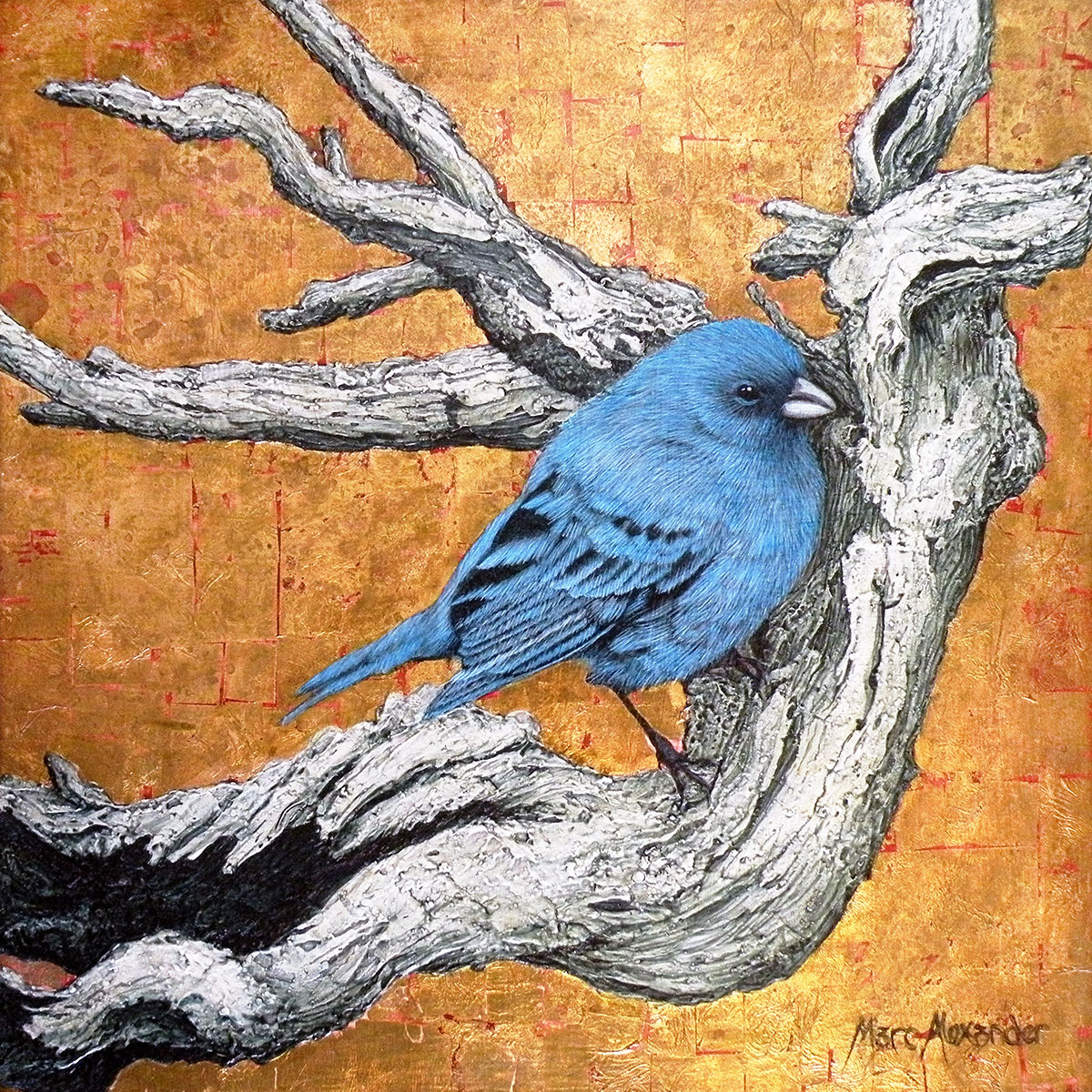##### Indigo Bunting
Currency conversion from USD to: [convert number=505.00 from="usd" to="zar"] ZAR | [convert number=505.00 from="usd" to="gbp"] GBP | [convert number=505.00 from="usd" to="eur"] EUR     Medium: Oil and Gold Leaf on Canvas Size: 30cm by 30cm SOLD
\$ 505.00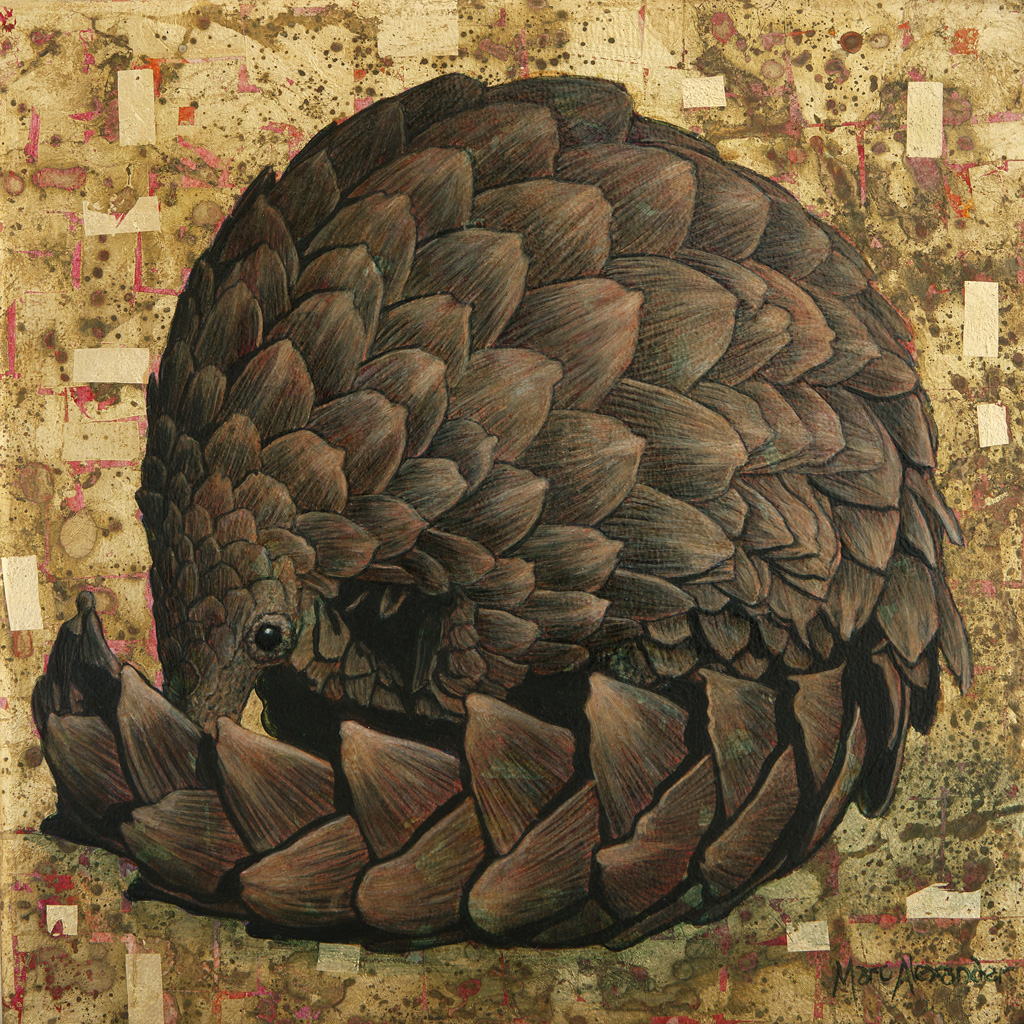##### Pangolin
Currency conversion from USD to: [convert number=650.00 from="usd" to="zar"] ZAR | [convert number=650.00 from="usd" to="gbp"] GBP | [convert number=650.00 from="usd" to="eur"] EUR     Medium: Oil and Gold Leaf on Canvas Size: 30cm by 30cm SOLD
\$ 650.00
Sale!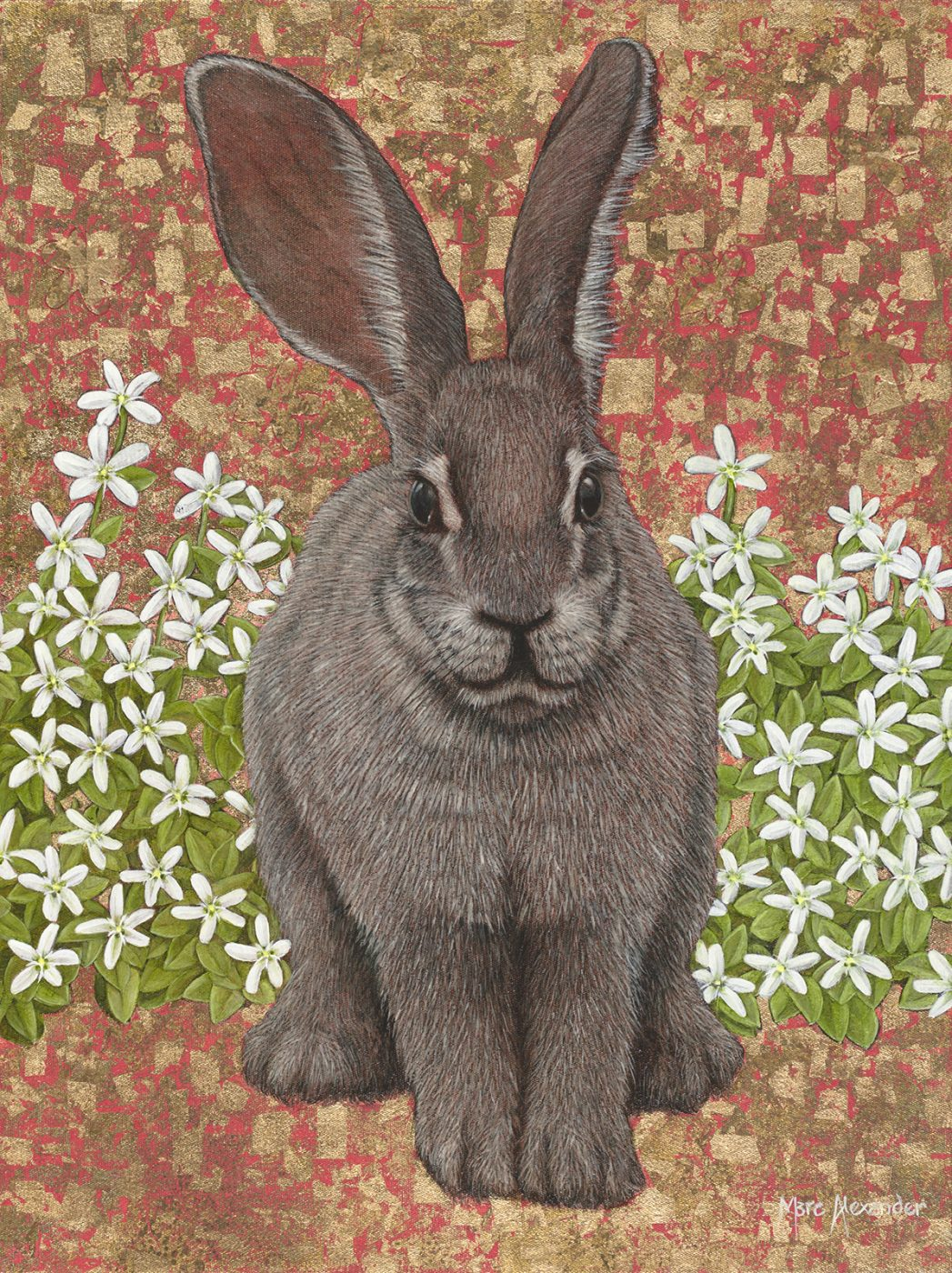##### Riverine Rabbit
Currency conversion from USD to: [convert number=700.00 from="usd" to="zar"] ZAR | [convert number=700.00 from="usd" to="gbp"] GBP | [convert number=700.00 from="usd" to="eur"] EUR     Medium: Oil and Gold Leaf on Canvas Size: 50cm by 40cm
\$ 1,350.00 \$ 700.00##### James and the Giant Tarantula
Currency conversion from USD to: [convert number=1350.00 from="usd" to="zar"] ZAR | [convert number=1350.00 from="usd" to="gbp"] GBP | [convert number=1350.00 from="usd" to="eur"] EUR     Medium: Oil and Gold Leaf on Canvas Size: 50cm by 40cm SOLD
\$ 1,350.00##### Ruby Forest
Currency conversion from USD to: [convert number=1350.00 from="usd" to="zar"] ZAR | [convert number=1350.00 from="usd" to="gbp"] GBP | [convert number=1350.00 from="usd" to="eur"] EUR     Medium: Oil and Gold Leaf on Canvas Size: 40cm by 540cm SOLD
\$ 1,350.00##### Snowy Owl
Currency conversion from USD to: [convert number=1400.00 from="usd" to="zar"] ZAR | [convert number=1400.00 from="usd" to="gbp"] GBP | [convert number=1400.00 from="usd" to="eur"] EUR     Medium: Oil and Gold Leaf on Canvas Size: 74cm by 50cm SOLD
\$ 1,400.00
Sale!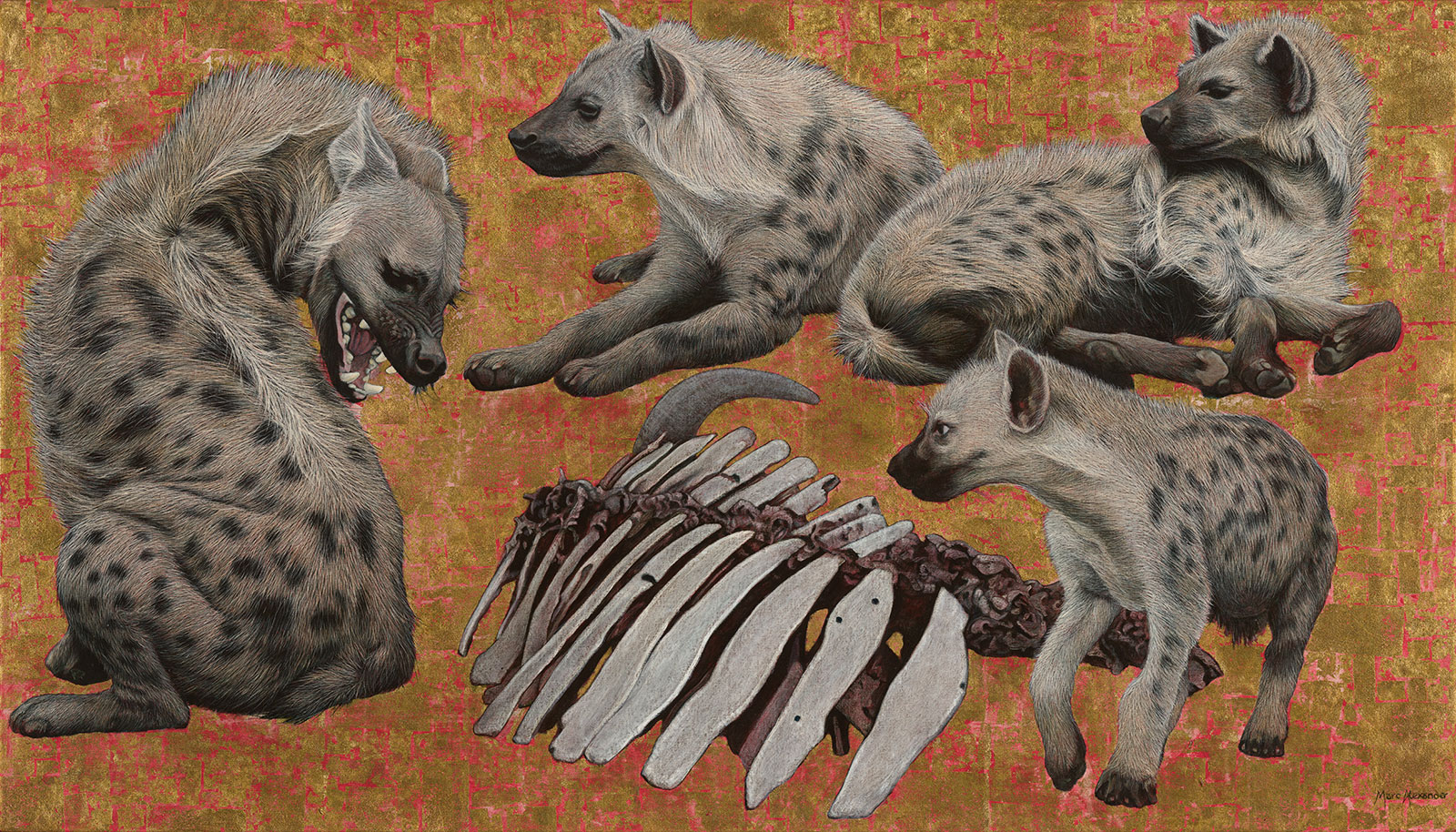##### Spotted Hyenas
Currency conversion from USD to: [convert number=1900.00 from="usd" to="zar"] ZAR | [convert number=1900.00 from="usd" to="gbp"] GBP | [convert number=1900.00 from="usd" to="eur"] EUR     Medium: Oil and Gold Leaf on Canvas Size: 80cm by 140cm
\$ 3,650.00 \$ 1,900.00
Sale!##### Enchanted Forest III
Currency conversion from USD to: [convert number=2200.00 from="usd" to="zar"] ZAR | [convert number=2200.00 from="usd" to="gbp"] GBP | [convert number=2200.00 from="usd" to="eur"] EUR     Medium: Oil and Gold Leaf on Canvas Size: 90cm by 120cm
\$ 3,950.00 \$ 2,200.00##### Himba Children
Currency conversion from USD to: [convert number=2200.00 from="usd" to="zar"] ZAR | [convert number=2200.00 from="usd" to="gbp"] GBP | [convert number=2200.00 from="usd" to="eur"] EUR     Medium: Oil and Gold Leaf on Canvas Size: 90cm by 120cm SOLD
\$ 2,200.00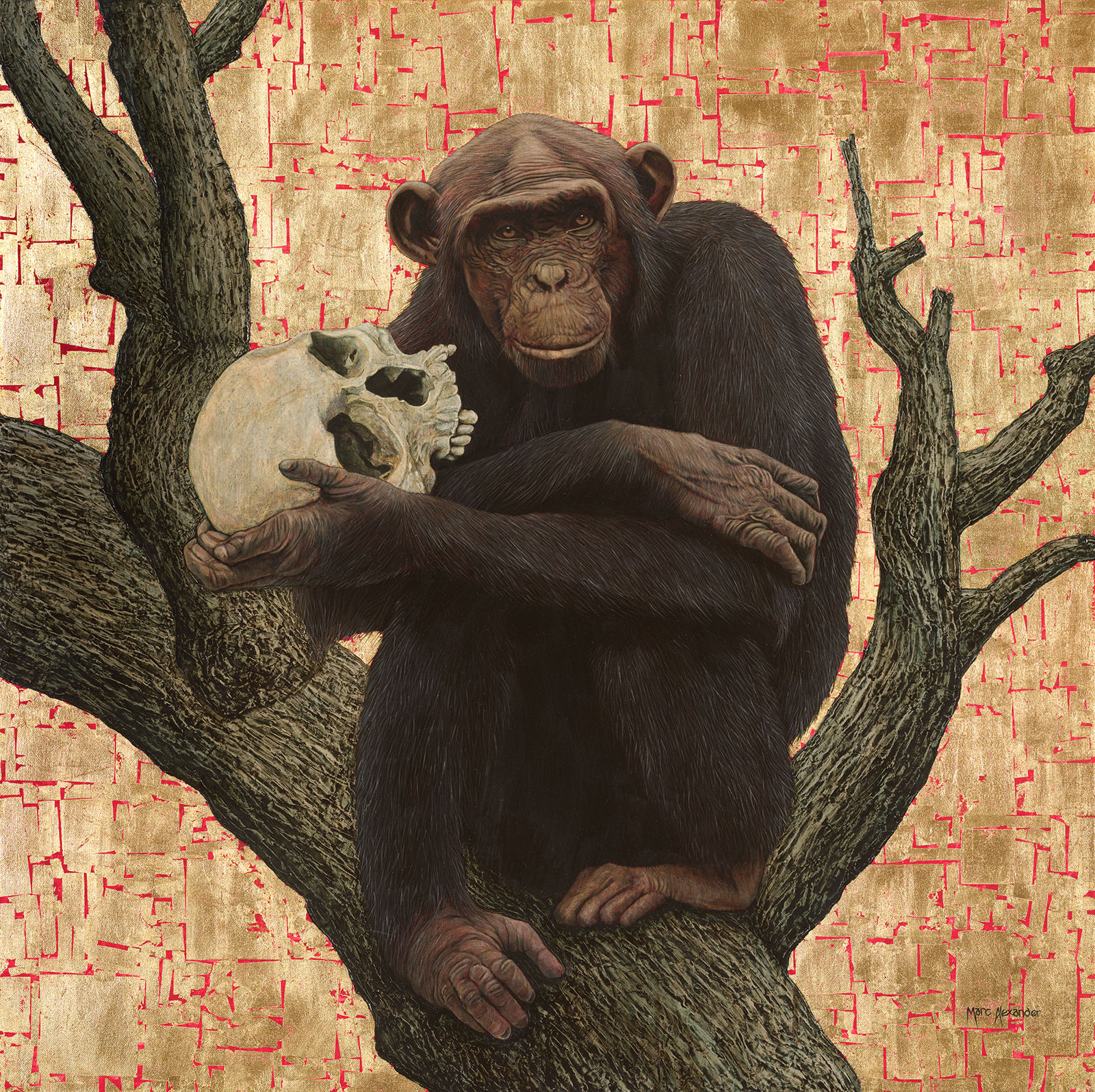##### Darwin’s Delusion
Currency conversion from USD to: [convert number=2250.00 from="usd" to="zar"] ZAR | [convert number=2250.00 from="usd" to="gbp"] GBP | [convert number=2250.00 from="usd" to="eur"] EUR     Medium: Oil and Gold leaf on Canvas Size: 100cm by 100cm SOLD
\$ 2,250.00##### Giraffe
Currency conversion from USD to: [convert number=2300.00 from="usd" to="zar"] ZAR | [convert number=2300.00 from="usd" to="gbp"] GBP | [convert number=2300.00 from="usd" to="eur"] EUR     Medium: Oil and Gold Leaf on Canvas Size: 100cm by 100cm SOLD
\$ 2,300.00##### Enchanted Forest II
Currency conversion from USD to: [convert number=2500.00 from="usd" to="zar"] ZAR | [convert number=2500.00 from="usd" to="gbp"] GBP | [convert number=2500.00 from="usd" to="eur"] EUR     Medium: Oil on Canvas Size: 100cm by 100cm SOLD
\$ 2,500.00##### African Buffalo
Currency conversion from USD to: [convert number=3450.00 from="usd" to="zar"] ZAR | [convert number=3450.00 from="usd" to="gbp"] GBP | [convert number=3450.00 from="usd" to="eur"] EUR     Medium: Oil and Gold Leaf on Canvas Size: 90cm by 120cm SOLD
\$ 3,450.00##### Bateleur Eagle I
Currency conversion from USD to: [convert number=3450.00 from="usd" to="zar"] ZAR | [convert number=3450.00 from="usd" to="gbp"] GBP | [convert number=3450.00 from="usd" to="eur"] EUR     Medium: Oil and Gold Leaf on Canvas Size: 90cm by 120cm SOLD
\$ 3,450.00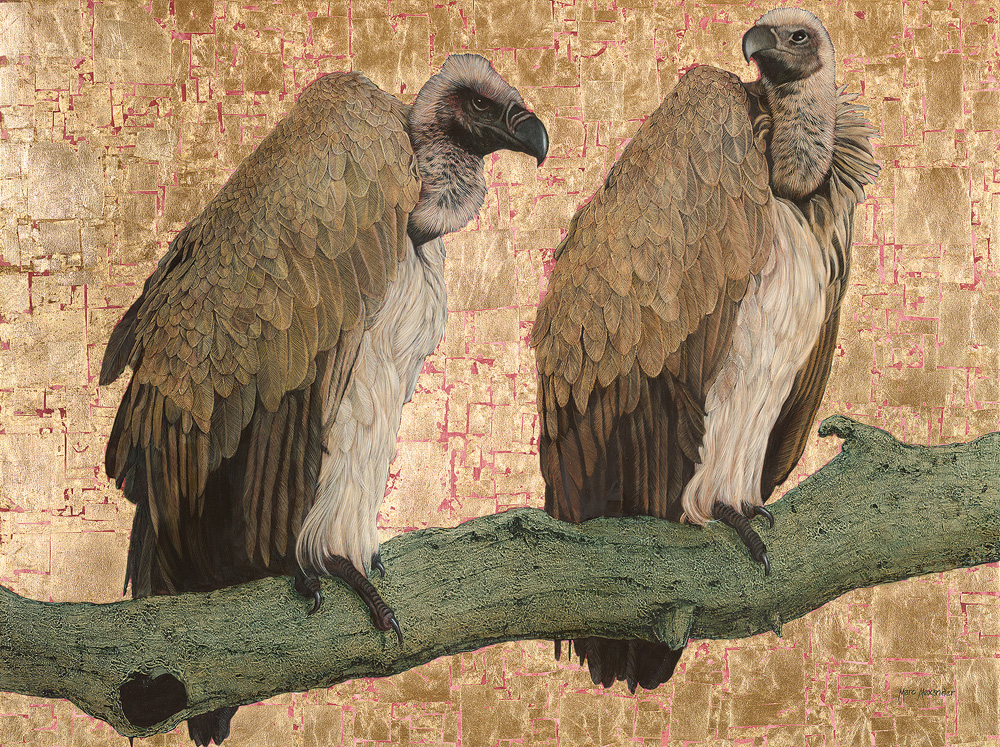##### Cape Vultures
Currency conversion from USD to: [convert number=3650.00 from="usd" to="zar"] ZAR | [convert number=3650.00 from="usd" to="gbp"] GBP | [convert number=3650.00 from="usd" to="eur"] EUR     Medium: Oil and Gold Leaf on Canvas Size: 90cm by 120cm SOLD
\$ 3,650.00##### Rhino II
Currency conversion from USD to: [convert number=3650.00 from="usd" to="zar"] ZAR | [convert number=3650.00 from="usd" to="gbp"] GBP | [convert number=3650.00 from="usd" to="eur"] EUR     Medium: Oil and Gold Leaf on Canvas Size: 90cm by 120cm SOLD
\$ 3,650.00##### Mighty Tusker
Currency conversion from USD to: [convert number=3650.00 from="usd" to="zar"] ZAR | [convert number=3650.00 from="usd" to="gbp"] GBP | [convert number=3650.00 from="usd" to="eur"] EUR     Medium: Oil and Gold Leaf on Canvas Size: 90cm by 120cm SOLD
\$ 3,650.00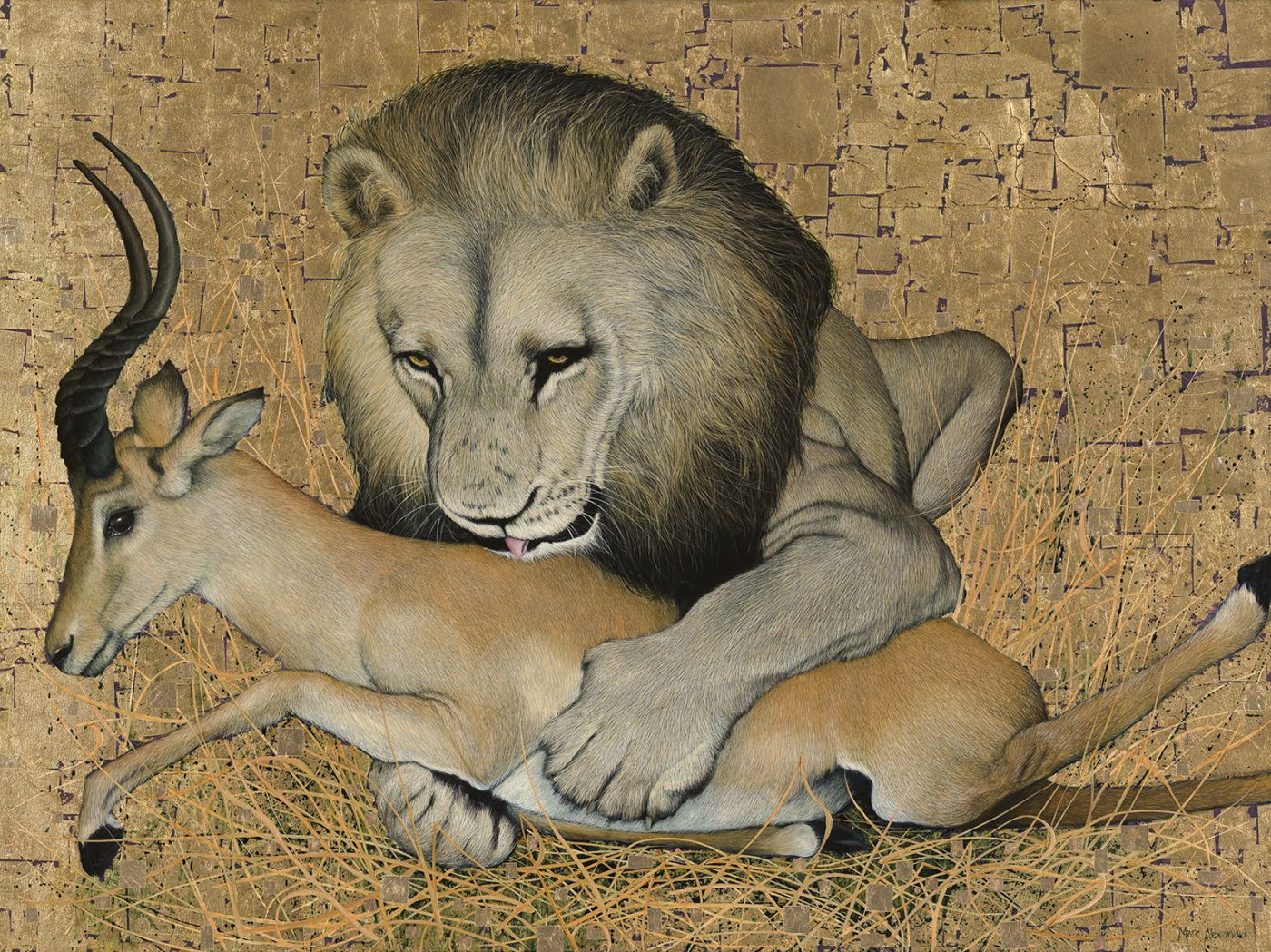##### The Kill
Currency conversion from USD to: [convert number=3650.00 from="usd" to="zar"] ZAR | [convert number=3650.00 from="usd" to="gbp"] GBP | [convert number=3650.00 from="usd" to="eur"] EUR     Medium: Oil and Gold Leaf on Canvas Size: 90cm by 120cm SOLD
\$ 3,650.00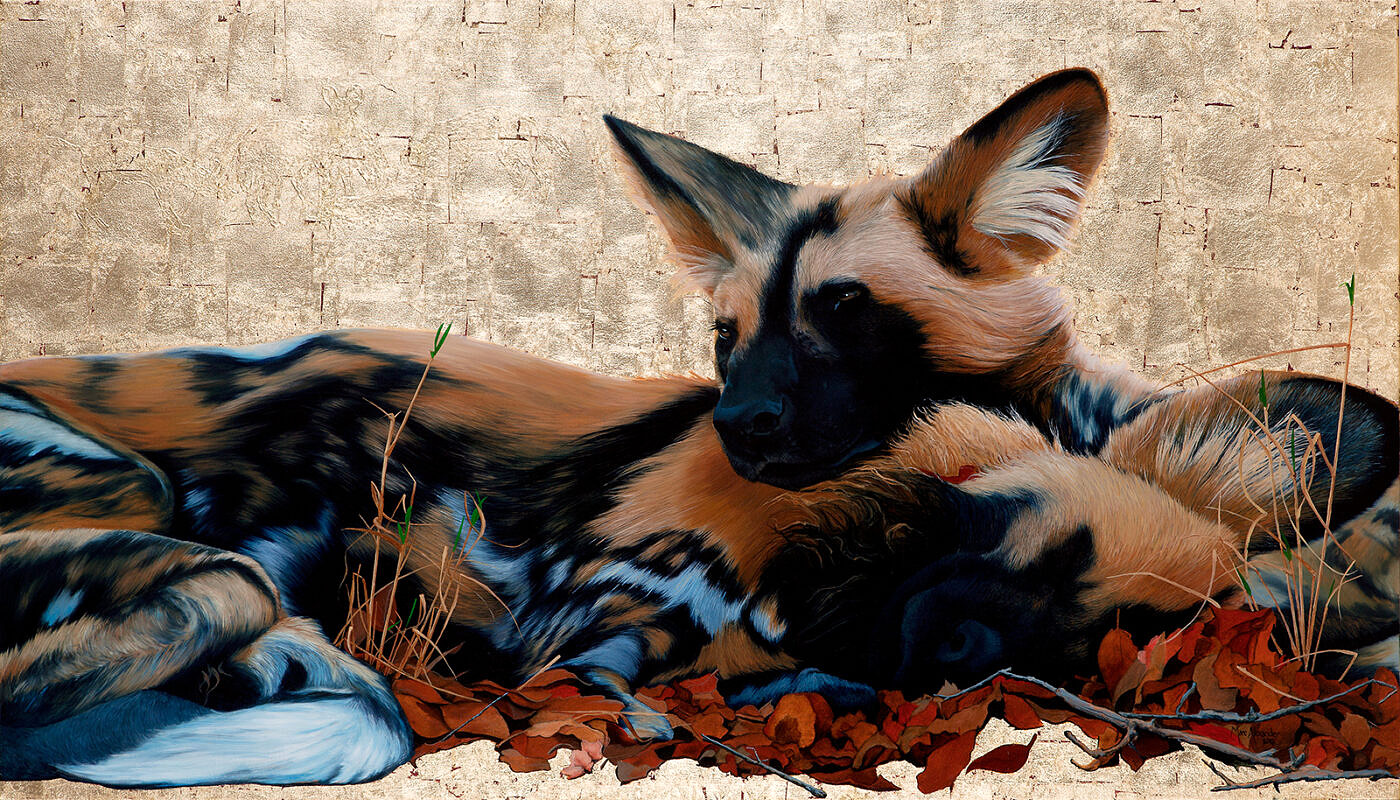##### Wild Dogs I
Currency conversion from USD to: [convert number=3650.00 from="usd" to="zar"] ZAR | [convert number=3650.00 from="usd" to="gbp"] GBP | [convert number=3650.00 from="usd" to="eur"] EUR     Medium: Oil and Gold Leaf on Canvas Size: 80cm by 140cm SOLD
\$ 3,650.00##### Wild Dogs at Dawn
Currency conversion from USD to: [convert number=3650.00 from="usd" to="zar"] ZAR | [convert number=3650.00 from="usd" to="gbp"] GBP | [convert number=3650.00 from="usd" to="eur"] EUR     Medium: Oil and Gold Leaf on Canvas Size: 80cm by 140cm SOLD
\$ 3,650.00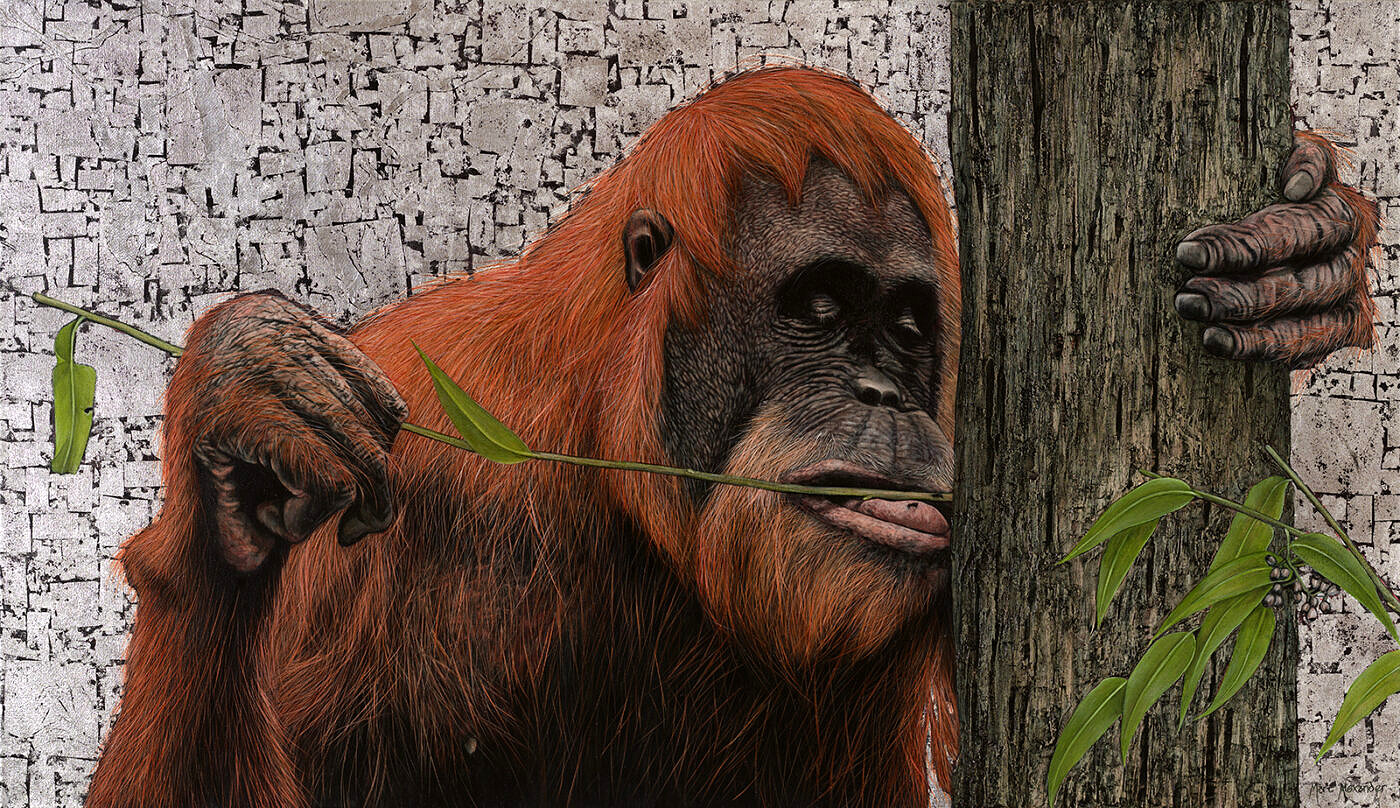##### Sumatran Orangutan
Currency conversion from USD to: [convert number=3650.00 from="usd" to="zar"] ZAR | [convert number=3650.00 from="usd" to="gbp"] GBP | [convert number=3650.00 from="usd" to="eur"] EUR     Medium: Oil and Silver Leaf on Canvas Size: 80cm by 140cm SOLD
\$ 3,650.00##### Bateleur Eagle II
Currency conversion from USD to: [convert number=3850.00 from="usd" to="zar"] ZAR | [convert number=3850.00 from="usd" to="gbp"] GBP | [convert number=3850.00 from="usd" to="eur"] EUR     Medium: Oil and Gold Leaf on Canvas Size: 120cm by 90cm SOLD
\$ 3,850.00